# The Arithmetic-Geometric Mean Iteration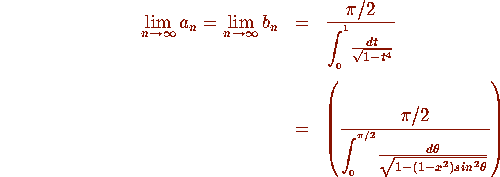In 1799, Gauss observed this purely numerically and wrote that this result

''will surely open a whole new field of analysis.''

# Equivalent Modular Parameterization

This is equivalent to the identities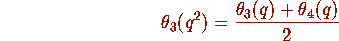and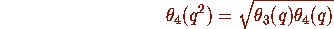where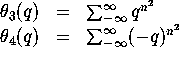These are modular forms. So for example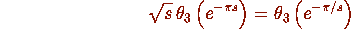# A Cubic Analogue of the AGM

Let

and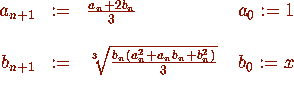then the common limit is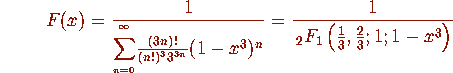The convergence is cubic.

## Proof

: The proof is opaque. It works because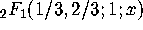satisfies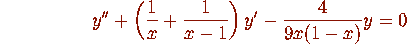In the above notation.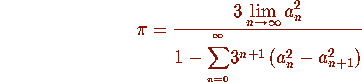where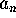is, as above, with,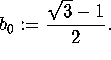Taking k+1 terms of the sum and limit gives a cubically convergent algorithm. Twenty one terms gives three billion digits.The underlying cubic transformation is beautiful: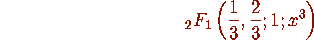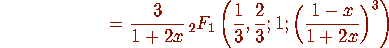# Equivalent Modular Parameterization

If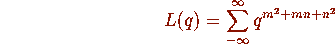and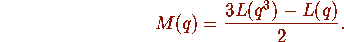Then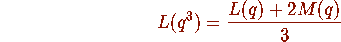and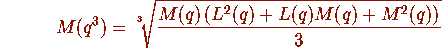Note that if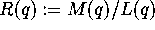then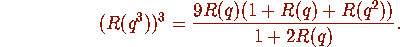This is a cubic modular equation for R.

See Some cubic modular identities of Ramanujan by J. Borwein, P. Borwein & F. Garvan in Trans. A.M.S. 343 (1994) 35-47.

# Some Explanations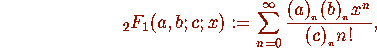where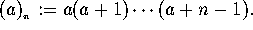Let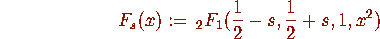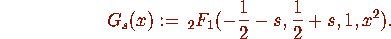Then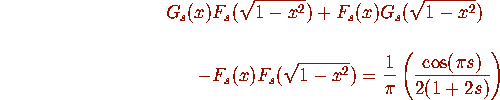and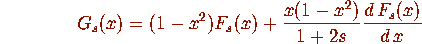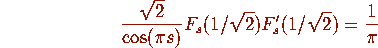If we can compute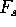iteratively we can compute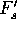and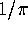by a second linked iteration.

There are four particularly interesting cases.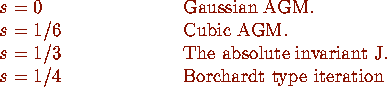Inverting the ratios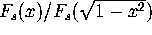gives elliptic modular functions. Which reduces much of this to an algebraic theory.

Finding and proving these iterations can (at least in principal) be effected entirely computationally.

# The Quadratic s=1/4 Iteration

Let and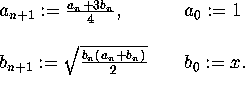Then the common limit is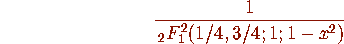# Caveat Emptor:

Let and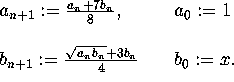Then the common limit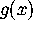is not differentially algebraic.